Courses

# Test: Extended Transition Function

## 10 Questions MCQ Test Theory of Computation | Test: Extended Transition Function

Description
This mock test of Test: Extended Transition Function for Computer Science Engineering (CSE) helps you for every Computer Science Engineering (CSE) entrance exam. This contains 10 Multiple Choice Questions for Computer Science Engineering (CSE) Test: Extended Transition Function (mcq) to study with solutions a complete question bank. The solved questions answers in this Test: Extended Transition Function quiz give you a good mix of easy questions and tough questions. Computer Science Engineering (CSE) students definitely take this Test: Extended Transition Function exercise for a better result in the exam. You can find other Test: Extended Transition Function extra questions, long questions & short questions for Computer Science Engineering (CSE) on EduRev as well by searching above.
QUESTION: 1

### The number of tuples in an extended Non Deterministic Finite Automaton:

Solution:

For NFA or extended transition function on NFA, the tuple elements remains same i.e. 5.

QUESTION: 2

### Choose the correct option for the given statement:Statement: The DFA shown represents all strings which has 1 at second last position   .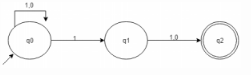Solution:

The given figure is an NFA. The statement contradicts itself.

QUESTION: 3

### What is wrong in the given definition?Def: ({q0, q1, q2}, {0,1}, δ, q3, {q3})

Solution:

q3 does not belong to Q where Q= set of finite states.

QUESTION: 4

If δ is the transition function for a given NFA, then we define the δ’ for the DFA accepting the same language would be:Note: S is a subset of Q and a is a symbol.

Solution:

According to subset construction, equation 1 holds true.

QUESTION: 5

What is the relation between DFA and NFA on the basis of computational power?

Solution:

DFA is said to be a specific case of NFA and for every NFA that exists for a given language, an equivalent DFA also exists.

QUESTION: 6

If a string S is accepted by a finite state automaton, S=s1s2s3……sn where siϵ∑ and there exists a sequence of states r0, r1, r2…… rn such that δ(r(i), si+1) =ri+1 for each 0, 1, …n-1, then r(n) is:

Solution:

r(n) is the final state and accepts the string S after the string being traversed through r(i) other states where I ϵ 01,2…(n-2).

QUESTION: 7

According to the given table, compute the number of transitions with 1 as its symbol but not 0: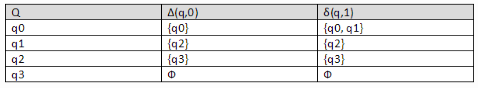Solution:

The transition graph is made and thus the answer can be found.

QUESTION: 8

From the given table, δ*(q0, 011) =?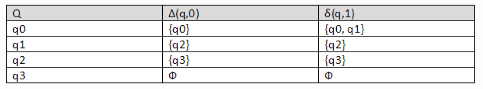Solution:

δ*(q0,011) = Uδ*(q0,01) δ (r, 1) = {q0, q1, q2}.

QUESTION: 9

Number of times the state q3 or q2 is being a part of extended 6 transition state is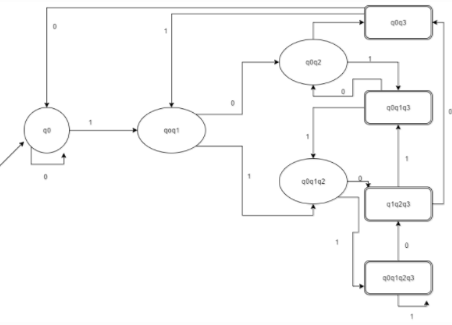Solution:

According to the question, presence of q2 or q1 would count so it does and the answer according to the diagram is 6.

QUESTION: 10

Predict the missing procedure: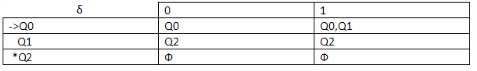1.Δ(Q0, ε) ={Q0},
2.Δ(Q0, 01) = {Q0, Q1}
3.δ(Q0, 010) =?

Solution:

According to given table and extended transition state implementation, we can find the state at which it rests.